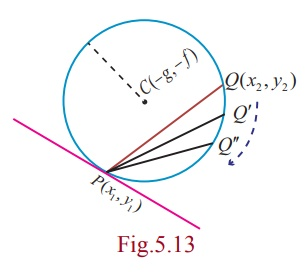Home | | Maths 12th Std | Equations of tangent and normal at a point P on a given circle

# Equations of tangent and normal at a point P on a given circle

Tangent of a circle is a line which touches the circle at only one point and normal is a line perpendicular to the tangent and passing through the point of contact.

Equations of tangent and normal at a point P on a given circle

Tangent of a circle is a line which touches the circle at only one point and normal is a line perpendicular to the tangent and passing through the point of contact.

Let P(x1 , y1 ) and Q(x2 , y2 ) be two points on the circle x2 + y2 + 2gx + 2 fy + c = 0 .Therefore,

x12 + y12 + 2gx1 + 2 fy1 + c = 0 ... (1)

and x22 + y22 + 2gx2 + 2fy2+ c = 0  ……..(2)

(2) - (1) givesTherefore, slope of PQ = - (x1 + x2 + 2g) / ( y1  + y2 + 2f )

When Q → P, the chord PQ becomes tangent at P

Slope  of tangent isHence, the equation of tangent is  y – y1Simplifying

yy1 + fy – y12 - fy1 + xx1 - x12 + gx - gx1 = 0

xx1  + yy1  + gx + fy - (x12  + y12  + gx1  + fy1 ) = 0 ...(1)

Since (x1 , y1 ) is a point on the circle, we have x12 + y12 + 2gx1 + 2 fy1 + c = 0

Therefore, -(x12 + y12 + gx + fy ) = gx1 + fy1 + c (2)

Hence, substituting (2) in (1), we get the equation of tangent at (x1 , y1 ) as

xx1 + yy1 + g(x + x1 ) + f ( y + y1 ) + c = 0 .

Hence, the equation of normal is

( y – y1 ) = [ ( y1 + f ) / ( x1  + g ) ] ( xx1 )

Þ ( y - y1 )( x1 + g ) = ( y1  + f )( x - x1 )

Þ x1 ( y - y1 ) + g ( y - y1 ) = y1 ( x - x1 ) + f ( x - x1 )

Þ yx1 - xy1 + g ( y - y1 ) - f ( x - x1 ) = 0 .

### Remark

(1) The equation of tangent at ( x1 , y1 ) to the circle x2+y2 = a2 is xx1 + yy1 = a2.

(2) The equation of normal at ( x1 , y1 ) to the circle x2+y2 = a2 is xy1 - yx1 = 0 .

(3) The normal passes through the centre of the circle.

Study Material, Lecturing Notes, Assignment, Reference, Wiki description explanation, brief detail
12th Mathematics : UNIT 5 : Two Dimensional Analytical Geometry II : Equations of tangent and normal at a point P on a given circle |